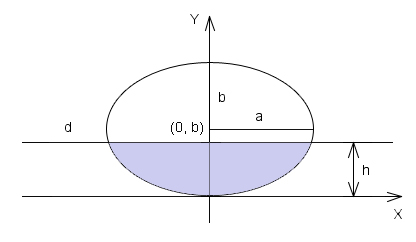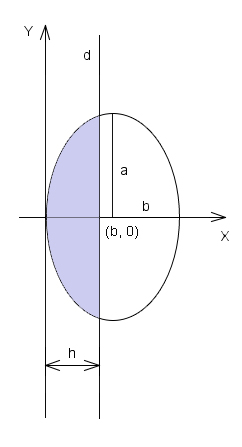•http://facebook.com/
•https://www.google.com/accounts/o8/id
•https://me.yahoo.com
COST (GBP)2.002.000

# ellipse

Computes the area of the ellipse segment determined by the intersection of an ellipse and a line parallel to the reference line.
Controller: CodeCogsContents## DependentsC++

## Ellipse

 doubleellipse( double a double b double h )[inline]
This module computes the area of the ellipse segment formed between an ellipse that is tangent to a reference line and another line parallel to this reference line, found at a given distance from it.

## Solution

Let us consider an ellipse with semiaxis a parallel to the reference line and semiaxis b perpendicular to this reference line, with the equation: The center of the ellipse is at . We also consider a line parallel to the reference line at a distance from it. The filled shape in the next image represents the area we must find.In order to compute the area by integration, we need to make the substitutions and , which makes the figure rotate clockwise. The filled shape in the next image represents half the area we must find.The new ellipse is tangent to the Y-axis and its center is found at , while the area of the shape remains the same after this coordinates transformation. In order to compute it we will first consider the equation of the equation of the new ellipse: with positive solution of the form:

### Example 1

#include <codecogs/maths/geometry/area/ellipse.h>
#include <stdio.h>

int main()
{
// the value of the height
double h = 0.973;

// the length of the semiaxes for the first ellipse (a>b)
double a1 = 1.925, b1 = 1.309;
// display the lenghts of the semiaxes for the first ellipse and the value of the height
printf("a1 = %.3lf\nb1 = %.3lf\nh = %.3lf\n\n", a1, b1, h);
// display the area for the first ellipse
printf("Area is %.5lf\n\n",Geometry::Area::ellipse(a1, b1, h));

// the length of the semiaxes for the second ellipse (a<b)
double a2 = 1.125, b2 = 2.345;
// display the lenghts of the semiaxes for the second ellipse and the value of the height
printf("a2 = %.3lf\nb2 = %.3lf\nh = %.3lf\n\n", a2, b2, h);
// display the area for the first ellipse
printf("Area is %.5lf\n",Geometry::Area::ellipse(a2, b2, h));

return 0;
}

Output:
a1 = 1.925
b1 = 1.309
h = 0.973

Area is 2.67888

a2 = 1.125
b2 = 2.345
h = 0.973

Area is 1.24346

### Parameters

 a semiaxis of the ellipse parallel to the reference line b semiaxis of the ellipse perpendicular to the reference line h the distance between line d and the reference line

### Returns

The area of the desired shape.

### Authors

Lucian Bentea, Eduard Bentea (September 2006)
##### Source Code

Source code is available when you buy a Commercial licence.

Not a member, then Register with CodeCogs. Already a Member, then Login.

Last Modified: 18 Dec 11 @ 23:00     Page Rendered: 2022-03-14 17:29:43